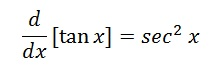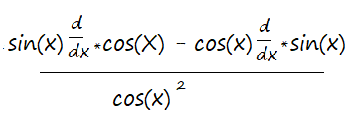# Derivative of Tan x & Proof in Easy Steps

Derivatives > Derivative of Tan x

## What is the Derivative of Tan x?

The derivative of tan x is sec2x:## How to Take the Derivative of Tan x

You can take the derivative of tan x using the quotient rule. That’s because of a basic trig identity, which is a quotient of the sine function and cosine function:

tan(x) = sin(x) / cos(x).

Step 1: Name the numerator (top term) in the quotient g(x) and the denominator (bottom term) h(x). You could use any names you like, as it won’t make a difference to the algebra. However, g(x) and h(x) are common choices.

• g(x) = sin(x)
• h(x) = cos(x)

Step 2: Put g(x) and h(x) into the quotient rule formula.Note that I used d/dx here to denote a derivative (Leibniz Notation) instead of g(x)′ or h(x)′ (Prime Notation (Lagrange), Function & Numbers). You can use either notation: they mean the same thing.

Step 3: Differentiate the functions from Step 2. There are two parts to differentiate:

1. The derivative of the first part of the function (sin(x)) is cos(x)
2. The derivative of cos(x) is -sin(x).

Placing those derivatives into the formula from Step 3, we get:Which we can rewrite as:
f′(x) = cos2(x) + sin2(x) / cos(x)2.

Step 4: Use algebra / trig identities to simplify.

• Specifically, start by using the identity cos2(x) + sin2(x) = 1
• This gives you 1/cos2(x), which is equivalent in trigonometry to sec2(x).

## Proof of the Derivative of Tan x

There are a couple of ways to prove the derivative tan x. You could start with the definition of a derivative and prove the rule using trigonometric identities. But there’s actually a much easier way, and is basically the steps you took above to solve for the derivative. As it relies only on trig identities and a little algebra, it is valid as a proof. Plus, it skips the need for using the definition of a derivative at all.

## Steps

Example problem: Prove the derivative tan x is sec2x.

Step 1: Write out the derivative tan x as being equal to the derivative of the trigonometric identity sin x / cos x:Step 2: Use the quotient rule to get:Step 3: Use algebra to simplify:Step 4: Substitute the trigonometric identity sin(x) + cos2(x) = 1:Step 5: Substitute the trigonometric identity 1/cos2x = sec2x to get the final answer:
d/dx tan x = sec2x
That’s it!

## References

Nicolaides, A. (2007). Pure mathematics: Differential calculus and applications, Volume 4. Pass Publications.

CITE THIS AS:
Stephanie Glen. "Derivative of Tan x & Proof in Easy Steps" From StatisticsHowTo.com: Elementary Statistics for the rest of us! https://www.statisticshowto.com/derivative-tan-x-proof/